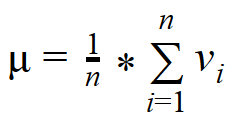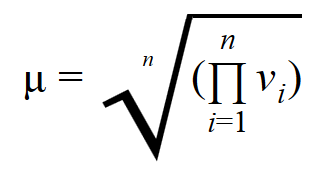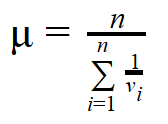- 12 minutes read

# SQL Statistical Analysis Part 2: Calculating Centers of Distribution

My previous article explained how to calculate frequencies using T-SQL queries. Frequencies are used to analyze the distribution of discrete variables. Today, we’ll continue learning about statistics and SQL. In particular, we’ll focus on calculating centers of distribution. We’ll learn e.g. how to calculate the SQL median, what functions to use to calculate the SQL mode, and how to calculate various types of mean in SQL (geometric mean, harmonic mean and, of course, arithmetic mean).

In statistics, certain measurements are known as moments. You can describe continuous variables (i.e. a variable that has a large range of possible numbers, such as household incomes in a country) with population moments. These moments give you insight into the distribution of the values (e.g. the percentile income levels) of continuous variables (e.g. the country’s income levels as a whole). The first four population moments include center, spread, skewness, and peakedness of distribution. In this article, we’ll focus on the first population moment: the center.

# What Is a Statistical Center?

If you think back to math class, you probably remember learning about the concept of averages. Roughly speaking, centers of distribution are like averages. Another way to think of them is as middle points in a range or sequence of values.

There are many ways to measure centers, but the three most popular ones should be familiar:

• The mode is the number that appears most often in a set of numbers. It is not necessarily unique—a distribution can have the same maximum frequency at different values. For example, in this grouping – 1, 4, 9, 8, 7, 7, 5, 7, 4, 3 – the number 7 appears the most. It is the mode.
• The median is the middle value in a distribution that has been sorted in increasing order. You might say that it splits the values into two halves: above the median and below it. When there is an odd number of values, the median is the middle entry in the sorted data. When there is an even number, the median is equal to the sum of the two middle numbers divided by two. In this grouping – 1, 2, 3, 4, 5, 6, 7 – the number 4 is the median. Note: There are types of medians, which I will explain when we discuss the T-SQL `PERCENTILE_CONT` and `PERCENTILE_DISC` functions.
• The arithmetic mean is the average value of a distribution. This is the familiar “average” that consists of the total sum of values divided by their number. For example, the arithmetic mean of 10, 20, 30, 40 is 25, or (10+20+30+40)/4.There are other types of means, such as the geometric mean or the harmonic mean. To avoid confusion, you should use the term arithmetic mean. However, for the sake of simplicity, I will use the term mean for the arithmetic mean; I’ll name the other two specifically when I show you how to calculate them.

# Why Use Multiple Center Measures?

It’s often useful to calculate more than one measure – more than one center of a distribution – if you want to understand the entire picture behind what the data is telling you. You can get some idea of the distribution just by comparing mode, median, and mean values. If the distribution is symmetrical and has only a single peak, then the mode, median, and mean will all coincide. If not, the distribution is skewed in some way.

How can a distribution be skewed? Perhaps it has a long tail to the right, like the distribution of household income. Then the mode would stay on the value with the highest relative frequency, but the median might move to the right to pick up half the observations. Half of the observations lie on either side of the median, but the cases on the right are farther out and exert more downward leverage. To balance them out, the mean must move even further to the right.

If the distribution of data is skewed to the left, the mean is less than the median, which is often less than the mode. If the distribution of data is skewed to the right, the mode is often less than the median, which is less than the mean. However, calculating how much the distribution is skewed means calculating another population moment, the skewness, which I will explain in another article.

## Data Used for Analyses

In this article, I am using pre-prepared data. Please check my previous article to learn the basics of data preparation for statistical analysis.

Once again, I am using the AdventureWorksDW2014 demo database. You can download a full backup of this database here. I am running all the code on SQL Server 2016 Developer Edition. I prefer to use the AdventureWorks sample database instead of SQL Server 2016’s WideWorldImportersDW sample database. The data in the WideWorldImportersDW database lacks the correlations and associations needed for statistical analysis.

# Calculating the Centers

It is time to start calculating the centers. We’ll look at each of the three common center measures individually.

## SQL Mode

The mode is the most fashionable value of a distribution— i.e. the one that appears the most. In fact, mode is actually the French word for fashion. Calculating the mode is simple and straightforward. In the following query, I use the `TOP (1) WITH TIES` expression to get the most frequently-appearing values in the distribution of the Age variable.

Note that multiple values could appear the same number of times. This means that a distribution could be multimodal. Because of that, I need to use the `WITH TIES` option of the `TOP` operator.

The following code calculates the mode for the Age variable from the `dbo.vTargetMail` view.

```USE AdventureWorksDW2014;
SELECT TOP (1) WITH TIES Age, COUNT(*) AS Number
FROM dbo.vTargetMail
GROUP BY Age
ORDER BY COUNT(*) DESC;
```

The code generates the following output:

```Age   Number
---   ------
35      704
```

You can see that the age of 35 is the most prevalent value and that there is only a single mode.

The `TOP` operator is not part of the ANSI SQL standard; it is a Transact-SQL extension. If you prefer to use standard SQL, the following query uses two common table expressions and the `RANK` window function to accomplish the same thing:

```WITH AgeCTE AS
(
SELECT Age, COUNT(*) AS Number
FROM dbo.vTargetMail
GROUP BY Age
),
AgeRankCTE AS
(
SELECT Age, Number,
RANK() OVER (ORDER BY Number DESC) AS AgeRank
FROM AgeCTE
)
SELECT Age, Number
FROM AgeRankCTE
WHERE AgeRank = 1;
```

This produces the same result as the T-SQL TOP query.

## SQL Median

The median is the value that splits the distribution into two halves. The number of rows that have values lower than the median must be equal to the number of rows that have values greater than the median. If there is an odd number of rows, the median is the middle row. If the number of rows is even, the median can be defined as the average value of the two middle rows (the financial median); the smaller of them (the lower statistical median), or the larger of them (the upper statistical median). The upper statistical median is very rarely used; many times, the lower statistical median is referred to as the statistical median.

### SQL Median: Calculating Lower Statistical Median

To calculate this type of the SQL median, you have to use the `PERCENTILE_DISC` function. It computes a specific percentile for sorted values in an entire rowset or within distinct partitions of a rowset. For the given percentile value P, `PERCENTILE_DISC` sorts the values of the expression in the `ORDER BY` clause and returns the value with the smallest `CUME_DIST` value (with respect to the same sort specification) that is greater than or equal to P. The `CUME_DIST` function calculates the cumulative distribution of a value in a group of values. Therefore, the `CUME_DIST` function computes the relative position of a specified value. `PERCENTILE_DISC` calculates the percentile based on a discrete distribution of the column values; the result is equal to a specific value in the column. In short, the PERCENTILE_DISC (0.5) function calculates the lower statistical median.

### SQL Median: Calculating Financial Median

The `PERCENTILE_CONT` function calculates a percentile based on a continuous distribution of the column value in SQL Server. The result is interpolated and might not be equal to any specific value in the column. Therefore, this function calculates another type of the SQL median: the financial median. It is by far the most used median.

The following code shows the difference between the `PERCENTILE_DISC` and `PERCENTILE_CONT` functions used for calculating the SQL median. It creates a simple table and inserts four rows with the values 1, 2, 3, and 4 in the only column in the table. Then it calculates the lower statistical and financial medians:

```IF OBJECT_ID('dbo.TestMedian','U') IS NOT NULL
DROP TABLE dbo.TestMedian;
GO
CREATE TABLE dbo.TestMedian
(
Val INT	NOT NULL
);
GO
INSERT INTO dbo.TestMedian (Val)
VALUES (1), (2), (3), (4);
SELECT DISTINCT			-- can also use TOP (1)
PERCENTILE_DISC(0.5) WITHIN GROUP (ORDER BY Val) OVER () AS MedianDisc,
PERCENTILE_CONT(0.5) WITHIN GROUP (ORDER BY Val) OVER () AS MedianCont
FROM dbo.TestMedian;
```

The code returns the following result:

```MedianDisc   MedianCont
----------   ----------
2          2.5
```

You can clearly see the difference between the two functions.

Now let’s use `PERCENTILE_CONT` to calculate the financial type of the SQL median for the Age column of the `dbo.vTargetMail` view.

```SELECT DISTINCT
PERCENTILE_CONT(0.5) WITHIN GROUP (ORDER BY Age) OVER () AS Median
FROM dbo.vTargetMail;
```

Here is the result:

```Median
------
45
```

You probably noticed that the median age (45) is bigger than the mode age (35). Before drawing any conclusions, let’s find the mean age.

## SQL Mean

The mean is the most common measure for determining the center of a distribution. It is also probably the most abused statistical measure. The mean does not mean anything without some other statistical measure, e.g. without the standard deviation, which I will explain in another article. It should never be used alone.

Let me give you an example. Imagine there are two pairs of people. In the first pair, both people earn the same salary of \$80,000 per year. In the second pair, one person earns \$30,000 per year and the other earns \$270,000 per year. The mean wage for the first pair is \$80,000, while the mean for the second pair is \$150,000 per year. By just listing the mean, you could conclude that each person in the second pair earns more than either of the people in the first pair. However, this would be a seriously incorrect conclusion.

The definition of the mean is simple: it is the sum of all values of a continuous variable divided by the number of cases, as shown in the following formula:Because of the great importance of the mean, T-SQL has the AVG aggregate function. The following query uses it to calculate the mean value for the Age variable:

```SELECT AVG(1.0*Age) AS Mean
FROM dbo.vtargetMail;
```

The mean value is shown in the following result:

```Mean
---------
47.515094
```

Note: In this query, I am multiplying Age by 1.0 to implicitly convert it from an integer to a decimal data type and get a decimal result.

Compare this value to the value for the SQL median and the SQL mode. The mean is higher than the median, which is higher than the mode. This means that the distribution of the Age variable is skewed to the right. It has a long tail of infrequent but high values on the right side—that is, on the side with bigger values.

The arithmetic mean is the first population moment. It is also called the estimated value or estimator because you can use it to estimate the value of a variable for an unknown case. However – and as you have already seen – the mean alone can be a very bad estimator, so when calculating the SQL mean, remember to provide other numbers as well.

## SQL Geometric Mean

The geometric mean is defined as the n-th root of the product of all values in the data, where there are n values. It is rarely used; I’d say that it is perhaps most appropriate for calculating the mean in a set of values which are exponentially distributed. The geometric mean applies only to numbers of the same sign, typically positive numbers. The formula, shown below, is quite simple:In fact, there is a problem with calculating the SQL geometric mean: SQL Server does not have a product aggregate function. With a little mathematical help, you can calculate the SQL geometric mean on your own. Just remember a few logarithm formulas:

```log(x*y) = log(x) + log(y)
exp(log(x*y)) = x*y
exp(log(x) + log(y)) = x*y
```

With this knowledge, you can use SQL Server’s `POWER` and `LOG10` mathematical functions and the standard SQL `SUM` and `COUNT` aggregate functions to calculate the SQL geometric mean. Just make sure to include the n-th root (as 1/n) inside the argument for the `POWER` function. This will prevent any numeric overflows that could happen if you calculated the product first and the n-th root of the product afterwards.

The following code shows how to do this:

```SELECT POWER(10.0000, SUM(LOG10(1.0*Age))/COUNT(*)) AS GeometricMean
FROM dbo.vtargetMail;
```

The result is:

```GeometricMean
-------------
46.1989
```

Because I used the `LOG10` function, this calculation only works on positive numbers.

## SQL Harmonic Mean

The harmonic mean is defined as the reciprocal of the arithmetic mean of the reciprocals of the values of a set. It is appropriate when dealing with rates and prices. Its use is limited, mostly to averaging rates. Here is the formula:Like the formula, the code for calculating the SQL harmonic mean is straightforward:

```SELECT COUNT(*)/SUM(1.0/Age) AS HarmonicMean
FROM dbo.vtargetMail;
```

The result is:

```HarmonicMean
-------------------
44.9695829524538739
```

Please note that the Age column is used as the denominator. This means that the code cannot work when the value of Age is zero.

# Conclusion

You can see the value of calculating multiple different measures for centers of a distribution. Comparing the SQL mode and the SQL median with the SQL mean gives you an idea of the skewness of a distribution. The higher population moments provide even more insights, but we’ll save those for future articles.

The other two centers introduced – SQL geometric mean and SQL harmonic mean – are used only for specific kinds of values and for very specific occasions. You should calculate both using positive values and only when you know they are the appropriate measures for the business problem you are solving.